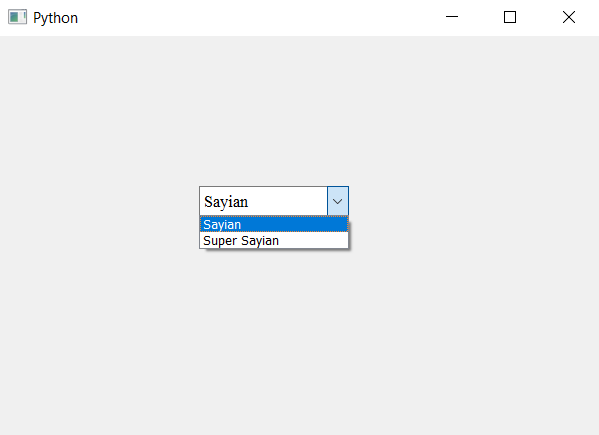# PyQt5 – Setting font to line editbox item of non-editable ComboBox

• Last Updated : 22 Apr, 2020

In this article we will see how to change the size and font of the item present in the editable box. If we use `setFont` method which takes QFont object as argument, using it with the check box object will change the font of all the items present in the list.

Note : When we get the line edit object from the combo box it will make the combo box there fore there is a need to make the line edit non editable

In order to change the font and size of only the item which is in the editable box, do the following –

1. Create a combo box
2. Get the line edit(editable box) object from combo box
3. Change the font of the line edit using `setFont` method
4. Make the line edit non-editable(read-only)

Below is the implementation –

 `# importing libraries``from` `PyQt5.QtWidgets ``import` `*` `from` `PyQt5 ``import` `QtCore, QtGui``from` `PyQt5.QtGui ``import` `*` `from` `PyQt5.QtCore ``import` `*` `import` `sys`` ` ` ` `class` `Window(QMainWindow):`` ` `    ``def` `__init__(``self``):``        ``super``().__init__()`` ` `        ``# setting title``        ``self``.setWindowTitle(``"Python "``)`` ` `        ``# setting geometry``        ``self``.setGeometry(``100``, ``100``, ``600``, ``400``)`` ` `        ``# calling method``        ``self``.UiComponents()`` ` `        ``# showing all the widgets``        ``self``.show()`` ` `    ``# method for widgets``    ``def` `UiComponents(``self``):`` ` `        ``# creating a combo box widget``        ``self``.combo_box ``=` `QComboBox(``self``)`` ` `        ``# setting geometry of combo box``        ``self``.combo_box.setGeometry(``200``, ``150``, ``150``, ``30``)`` ` `        ``# geek list``        ``geek_list ``=` `[``"Sayian"``, ``"Super Sayian"``]`` ` `        ``# making it editable``        ``self``.combo_box.setEditable(``True``)`` ` `        ``# adding list of items to combo box``        ``self``.combo_box.addItems(geek_list)`` ` `        ``# font``        ``font ``=` `QFont(``'Times'``, ``10``)`` ` `        ``# getting the line edit of combo box``        ``line_edit ``=` `self``.combo_box.lineEdit()`` ` `        ``# setting line font to the line edit``        ``line_edit.setFont(font)`` ` `        ``# making line edit non editable``        ``line_edit.setReadOnly(``True``)`` ` `# create pyqt5 app``App ``=` `QApplication(sys.argv)`` ` `# create the instance of our Window``window ``=` `Window()`` ` `# start the app``sys.exit(App.``exec``())`

Output :My Personal Notes arrow_drop_up